## Thursday, February 24, 2011

### Support vector machines (SVMs) in F# using Microsoft Solver Foundation

Support vector machines are a super star in machine learning and data mining in the past decade. It reheats statistical learning in machine learning community. It is also one of the classifiers that work practically with applications to other disciplines such as bioinformatics.

In this post, I will give a brief review of the theory of this classifier and show an F# implementation using the quadratic programming (QP) solver in Microsoft Solver Foundation (MSF).

It may seem dull to start with mathematics. But the math in SVMs is actually quite intuitive and would be quite easy if one has some training in linear algebra and calculus. Also understanding the intuition behind the formulae is more important in the formulation itself. There are quite a lot of good SVM tutorials written by researchers. Thus I only write the important formulas that occur in the implementation. The reader could link the F# code with formulas directly.

My note is based on the machine learning course by Prof. Jaakkola [Jaakkola] and the Matlab implementation therein. The course material is highly comprehensive yet accurate.

## Background review for SVMs

Given a binary labeled dataset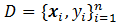, where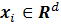and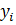is 1 or -1. Think about the data pointsplotted in a d dimensional (Euclidean) space, the linear SVM classifier is a hyperplane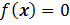in the space and it “best” separates the two kinds of data points. Here “best” means that the hyperplane should have the largest margin, which is the distance from the plane to the sides of labeled points. Intuitively the larger the margin is, the more robust and confident the classifier is. As if the hyperplane shakes a little, it still classifies well for its being far from both sides.

Let’s take d=2 as the discussing example: data points are plotted in a 2-D plane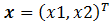. The aim is to draw a lineto separate the two kinds of points such that the margin of the separation is maximized. In the following Figure, the line with maximum margin is shown. After some geometry work, the margin is calculated as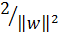, thus minimizing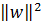with the constraints that the two kinds of points are well separated () gives the max-margin hyperplane.(Wikipedia picture)

Two important extensions to the above simplest SVM are:

1. Allowing imperfect separation, that is when a hyperplane could not separate the points perfectly, it is allowed to mis-separate some data points. This introduces a slack variablefor each data point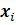, when the point is on the correct side of the plane, the slack variable is 0, otherwise it is measures the distance it goes from the plane minus 1, if the values goes to below zero set it zero. (Please read the Note and reference section for more explanation.)

2. Kernel. The simplest SVM is a linear classifier in the original space. However, there are situations where linear classifier is not sufficient (even the best), one strategy is to do a non-linear transformation or mapping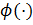that maps a data pointto another space, so that a linear classifier in that space, which is non-linear in the original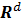space, does a better separation of the data points. The kernel trick is that we don’t need to defineexplicitly; only the definition of inner product of that space is required: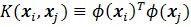.

With the two extensions, the new maximum margin objective becomes: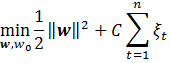subject to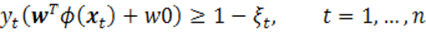The dual form: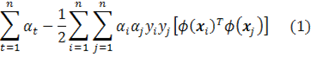subject to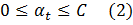The data points with its alpha value greater than 0 are called support vectors.

With the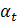in the dual problem solved, the SVM classification hyperplane is recovered by: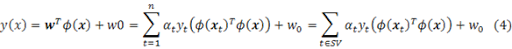The threshold parameter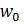could be calculated by substituting back to the support vectors: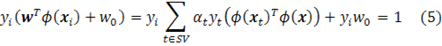## The implementation

The solver is actually an Interior Point Solver, which could solve linear and quadratic programming with linear constraints.

Page 14-17 of MSF-SolverProgrammingPrimer.pdf show an example usage of the QP solver in Microsoft Solver Foundation. But the example is not complete and it does not demonstrate one very subtle part: how to set the coefficients for the quadratic terms.

The following code example shows a simple example with the the comment regarding the coefficient setting:

Example usage of the QP solver
1. #r @"C:\Program Files (x86)\Sho 2.0 for .NET 4\packages\Optimizer\Microsoft.Solver.Foundation.dll"
2. open Microsoft.SolverFoundation.Common
3. open Microsoft.SolverFoundation.Solvers
4. open Microsoft.SolverFoundation.Services
5. // test QP
6. module TEST =
7. (* minimize x^2 + y^2 + 3xy + 2x + y *)
8. (* notice that in the InteriorPointSolver,
9. the coefficients for xy & yx should be the same (so only set ONCE!)
10. if we set both 3xy and 0yx, the solver takes the later coef.
11. *)
12. let solver = new InteriorPointSolver()
13. let _, goal = solver.AddRow("dual objective value")
14. solver.AddGoal(goal, 0, true)
15. let _, x = solver.AddVariable("x")
16. let _, y = solver.AddVariable("y")
17. solver.SetCoefficient(goal, x, Rational.op_Implicit(2))
18. solver.SetCoefficient(goal, y, Rational.op_Implicit(1))
19. // for terms like x-y (where x != y), set its coef for only one time!
20. solver.SetCoefficient(goal, Rational.op_Implicit(3), x, y)
21. //solver.SetCoefficient(goal, Rational.Zero, y, x)
22. solver.SetCoefficient(goal, Rational.op_Implicit(1), x, x)
23. solver.SetCoefficient(goal, Rational.op_Implicit(1), y, y)
24. let param = new InteriorPointSolverParams()
25. solver.Solve(param) |> ignore
26. //solver.Result
27. let objectiveValue = solver.GetValue(0).ToDouble()
28. let x0 = solver.GetValue(1).ToDouble()
29. let y0 = solver.GetValue(2).ToDouble()
30. x0*x0 + y0*y0 + 3.0 * x0 * y0 + 2. * x0 + y0
31. //x0*x0 + y0*y0 + 0.0 * x0 * y0 + 2. * x0 + y0

The implementation of SVM is a straightforward translation of equations (1) to (5). The following shows the svm learning (bulidSvm) and testing (svmClassify) functions:

SVM Implementation
1. open System
2. type dataset =
3. { features: float array array; // (instance = float array) array
4. mutable labels: int array; //
5. }
6. with
7. member x.NumSamples = x.features.Length
8. module Array =
9. let median (a:'a array) =
10. let sorted = Array.sort a
11. sorted.[sorted.Length / 2]
12. module Kernel =
13. let linear a b =
14. Array.fold2 (fun acc p q -> acc + p * q) 0.0 a b
15. let polynomial k a b =
16. let dot = linear a b
17. Math.Pow(1.0 + dot, k |> float)
18. let gaussian beta a b =
19. let diff = Array.fold2 (fun acc p q -> acc + (p-q)*(p-q)) 0.0 a b
20. exp (-0.5 * beta * diff)
21. module SVM =
22. type svmmodel = {
23. SVs:dataset;
24. alpha:float array;
25. kernel: float[] -> float[] -> float;
26. w0:float;
27. }
28. with
29. member x.NumSupporVectors = x.SVs.features.Length
30. let buildSVM (ds:dataset) (C:float) (kernel:float[] -> float[] -> float) =
31. let n = ds.features.Length
32. let C = Rational.op_Implicit(C)
33. let zero = Rational.Zero
34. // create a interior point solver, which solves the QP problem
35. let solver = new InteriorPointSolver()
36. // set the objective value / goal
37. let _, goal = solver.AddRow("dual objective value")
38. // false == maximizing the objective value
39. // the value of goal is (1)
40. solver.AddGoal(goal, 0, false) |> ignore
41. // add the Lagangian variables \alpha_i and set their bounds (0 <= \alpha_i <= C)
42. let alpha = Array.create n 0
43. for i=0 to n-1 do
44. let _, out = solver.AddVariable("alpha_"+i.ToString())
45. alpha.[i] <- out
46. solver.SetBounds(out, zero, C)
47. // add contraint: \sum_i \alpha_i * y_i = 0
48. // equation (2)
49. let _, sumConstraint = solver.AddRow("SumConstraint")
50. solver.SetBounds(sumConstraint, zero, zero);
51. for i=0 to n-1 do
52. // set the coefs for the sum constraint
53. // equation (2)
54. solver.SetCoefficient(sumConstraint, alpha.[i], Rational.op_Implicit(ds.labels.[i]))
55. // add the \alpha_i terms into the objective
56. solver.SetCoefficient(goal, alpha.[i], Rational.One)
57. // add the qudratic terms
58. for j=0 to i do
59. // coef = y_i * y_j * K(x_i, x_j)
60. let coef = float(ds.labels.[i] * ds.labels.[j]) * (kernel ds.features.[i] ds.features.[j])
61. if i=j then
62. solver.SetCoefficient(goal, Rational.op_Implicit(-0.5 * coef), alpha.[i], alpha.[j])
63. else
64. solver.SetCoefficient(goal, Rational.op_Implicit(-coef), alpha.[i], alpha.[j])
65. // use the default parameters
66. let param = new InteriorPointSolverParams()
67. solver.Solve(param) |> ignore
68. // get the alpha values out
69. let alphaValue = Array.init n (fun i -> solver.GetValue(i+1))
70. (* print optimization result
71. printfn "goal value = %A" (solver.GetValue(0).ToDouble())
72. for i=1 to n do
73. printfn "%A" (solver.GetValue(i).ToDouble())
74. *)
75. let alphaNew = new ResizeArray<Rational>()
76. // extract the non-zero alpha values out and their corresponding support vectors
77. let SVs =
78. let feats = new ResizeArray<float[]>()
79. let labs = new ResizeArray<int>()
80. let maxAlpha = Array.max alphaValue
81. let threshold = maxAlpha * Rational.op_Implicit(1e-8)
82. for i=0 to n-1 do
83. if alphaValue.[i] > threshold then
87. { features = feats |> Seq.toArray;
88. labels = labs |> Seq.toArray;
89. }
90. // solve w_0 in the primal form
91. let alphaNZ = alphaNew |> Seq.toArray
92. // equation (5)
93. let w0 =
94. alphaNZ
95. |> Array.mapi (fun i a ->
96. if a = C then
97. None
98. else
99. let mutable tmp = 0.0
100. for j=0 to SVs.NumSamples-1 do
101. tmp <- tmp + alphaNZ.[j].ToDouble() * (SVs.labels.[j] |> float) * (kernel SVs.features.[i] SVs.features.[j])
102. Some ((float SVs.labels.[i]) - tmp)
103. )
104. |> Array.filter (fun v -> match v with None -> false | _ -> true)
105. |> Array.map (fun v -> match v with Some v -> v | _ -> 0.0)
106. |> Array.median
107. // construct an svm record
108. {
109. SVs = SVs;
110. alpha = alphaNZ |> Array.map (fun v -> v.ToDouble());
111. kernel = kernel;
112. w0 = w0;
113. }
114. let svmClassify (model:svmmodel) (ds:dataset) =
115. // equation (4)
116. let vals =
117. ds.features
118. |> Array.map (fun x ->
119. let mutable sum = 0.0
120. for i=0 to model.NumSupporVectors-1 do
121. sum <- sum + model.alpha.[i] * (float model.SVs.labels.[i]) * (model.kernel model.SVs.features.[i] x)
122. sum + model.w0
123. )
124. let nCorrect =
125. Array.map2 (fun value label -> if (value > 0.0) && (label = 1) || (value < 0.0) && (label = -1) then 1 else 0) vals ds.labels
126. |> Array.sum
127. (float nCorrect) / (float ds.NumSamples), vals

Try on the iris data set we used in the Logistic Regression post:

let svm = buildSVM iris 10.0 Kernel.linear
let classifyResult = svmClassify svm iris

val svm : svm =
{SVs =
{features =
[|[|1.0; 6.2; 2.2; 4.5; 1.5|]; [|1.0; 5.9; 3.2; 4.8; 1.8|];
[|1.0; 6.3; 2.5; 4.9; 1.5|]; [|1.0; 6.7; 3.0; 5.0; 1.7|];
[|1.0; 6.0; 2.7; 5.1; 1.6|]; [|1.0; 5.4; 3.0; 4.5; 1.5|];
[|1.0; 4.9; 2.5; 4.5; 1.7|]; [|1.0; 6.0; 2.2; 5.0; 1.5|];
[|1.0; 6.3; 2.7; 4.9; 1.8|]; [|1.0; 6.2; 2.8; 4.8; 1.8|];
[|1.0; 6.1; 3.0; 4.9; 1.8|]; [|1.0; 6.3; 2.8; 5.1; 1.5|];
[|1.0; 6.0; 3.0; 4.8; 1.8|]|];
labels = [|-1; -1; -1; -1; -1; -1; 1; 1; 1; 1; 1; 1; 1|];};
alpha =
[|6.72796421; 10.0; 10.0; 10.0; 10.0; 6.475497298; 1.490719712;
8.547262902; 3.165478894; 10.0; 10.0; 10.0; 10.0|];
kernel = <fun:svm@226-32>;
w0 = -13.63716815;}

>
Real: 00:00:00.002, CPU: 00:00:00.000, GC gen0: 0, gen1: 0, gen2: 0

val classifyResult : float * float [] =
(0.97,
[|-2.787610618; -2.380530973; -1.42477876; -2.929203541; -1.681415929;
-1.96460177; -1.24778761; -6.106194692; -2.761061946; -2.973451328;
…. 4.203539824; 1.82300885|])

## Notes and references

The optimization in SVMs could be treated as a general QP problem as shown in our implementation. However, when the number of the data points is big, the QP grows too big to handle by the QP solver.

As a special QP problem, SVM has a lot of novel solutions proposed in the machine learning research area, e.g. the SMO approach [Platt] in LibSVM implementation and the Ball Vector Machine approach [Tsang] transforming the special QP into a (discrete) computational geometry problem, minimum enclosing ball. For practical usage of SVM, one usually uses these dedicated approaches.

Besides the maximum margin interpretation, SVMs also have theory roots in functional regularization theory, in which the optimizationis viewed as minimizing the error termwhile controlling the complexity of the function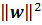and C is a tradeoff parameter between these two. The reader is referred to the teaching notes and papers by Prof. Poggio [Poggio].

[Burges] A Tutorial on Support Vector Machines for Pattern Recognition. http://research.microsoft.com/en-us/um/people/cburges/papers/svmtutorial.pdf

[Jaakkola] http://courses.csail.mit.edu/6.867/.

[Platt] Sequential Minimal Optimization: A Fast Algorithm for Training Support Vector Machines. ftp://ftp.research.microsoft.com/pub/tr/tr-98-14.pdf

[Poggio] http://www.mit.edu/~9.520/spring10/.

1.This comment has been removed by the author.

1.The development of artificial intelligence (AI) has propelled more programming architects, information scientists, and different experts to investigate the plausibility of a vocation in machine learning. Notwithstanding, a few newcomers will in general spotlight a lot on hypothesis and insufficient on commonsense application. machine learning projects for final year In case you will succeed, you have to begin building machine learning projects in the near future.

Projects assist you with improving your applied ML skills rapidly while allowing you to investigate an intriguing point. Furthermore, you can include projects into your portfolio, making it simpler to get a vocation, discover cool profession openings, and Final Year Project Centers in Chennai even arrange a more significant compensation.

Data analytics is the study of dissecting crude data so as to make decisions about that data. Data analytics advances and procedures are generally utilized in business ventures to empower associations to settle on progressively Python Training in Chennai educated business choices. In the present worldwide commercial center, it isn't sufficient to assemble data and do the math; you should realize how to apply that data to genuine situations such that will affect conduct. In the program you will initially gain proficiency with the specialized skills, including R and Python dialects most usually utilized in data analytics programming and usage; Python Training in Chennai at that point center around the commonsense application, in view of genuine business issues in a scope of industry segments, for example, wellbeing, promoting and account.

The Nodejs Projects Angular Training covers a wide range of topics including Components, Angular Directives, Angular Services, Pipes, security fundamentals, Routing, and Angular programmability. The new Angular TRaining will lay the foundation you need to specialise in Single Page Application developer. Angular Training

2.This comment has been removed by the author.

3.Great post Yin!

I was looking for info on how to use Microsoft Solver Foundation and I stumbled on your great blog again :)

4."one _tragedy_ is to do a non-linear transformation" ? I think the spell correction got the better of your text ;-)

"from the plane minus 1" - I hadn't seen that in my previous reading about SVMs. Isn't the slack just the distance past the boundary (without the -1)?

5.The SVM paper I was looking at was http://scribblethink.org/Work/Notes/svmtutorial.pdf, which on second reading does have the step that changes from a 0 to 1 slack excess.

6.@philipoakley: Thanks for the correction on the spelling. "from the plane minus 1" -- please search "hinge loss function" to get more details of it:)

7.The blue line is the hinge loss, which is 0 when the difference is below 1.

8.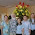This comment has been removed by the author.

9.March 2013
Hi Yin.
Thanks for your blogs on F# MSF.

What is the latest status of F# and MSF?

Thanks, Art Scott

10.Awesome post, Call our Team member at Microsoft Online Support Phone Number Canada and at the other hand Microsoft Support Number Canada at 1-888-582-4887 for instant help and support if you have any issue with MS Window.
Microsoft Office Support Canada 1-888-582-4887

11.12.they are real people. Its not like they are computer parts or something. cursos de ti online

13.14.15.16.17.18.19.20.21.22.23.Interesting Article. Hoping that you will continue posting an article having a useful information. Betnano giriş adresi

24.